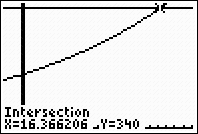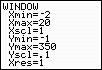Solving Exponential Equations While exponential equations can be solved on the graphing calculator, you will, in most cases, have to adjust your window to see the graphs.
 1.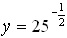Enter the right hand side of the equation on the home screen to perform the calculation.  Notice the use of parentheses. Answer:  y = .2  or  1/5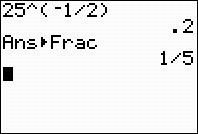2.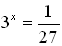Enter the left side into Y1 and the right side into Y2.   Keep in mind that 1/27 is a very small value.  Adjust your window accordingly.  If necessary, start with the standard 10 x 10 window and adjust until you see the graph clearly.  Then use your intersect option. 2nd CALC (above TRACE), #5 intersect Answer: x = -3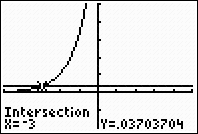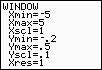3.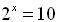Proceed as in #2.  A standard 10 x 10 window was used here. Answer:  x = 3.3219281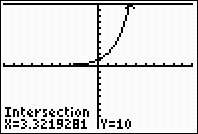4.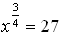Proceed as in #2.  Be sure to adjust the window to allow for a y-value of 27.  Also notice the need to move to the right to find the intersection.  Increase the XMax value. Answer:  x = 81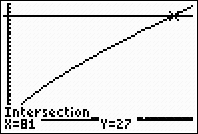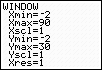5.    Solve this system: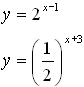Proceed as in #2.  Use the intersect option.  A standard 10 x 10 window was used here. Answer:  x = -1,  y = 0.25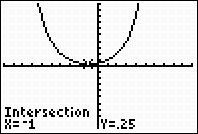6.  Solve for x, to the nearest thousandth: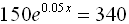Proceed as in #2.  Adjust the window as to see the point of intersection of the two functions. Answer:  x = 16.366